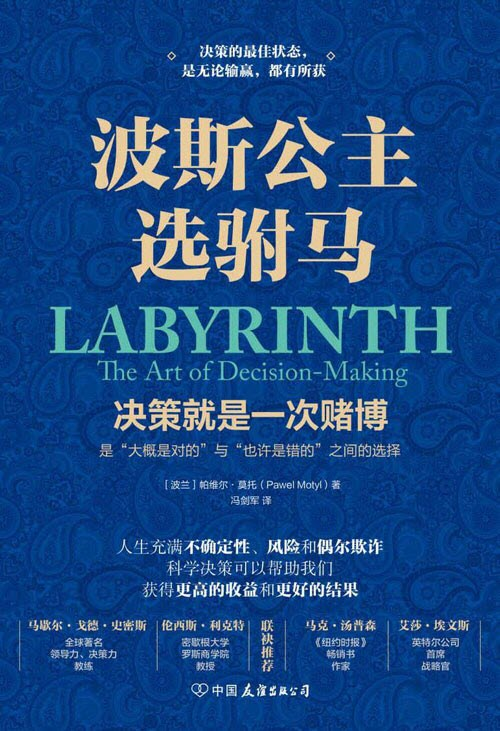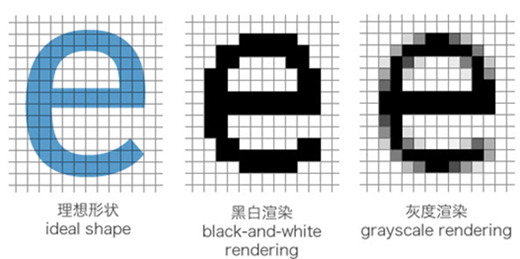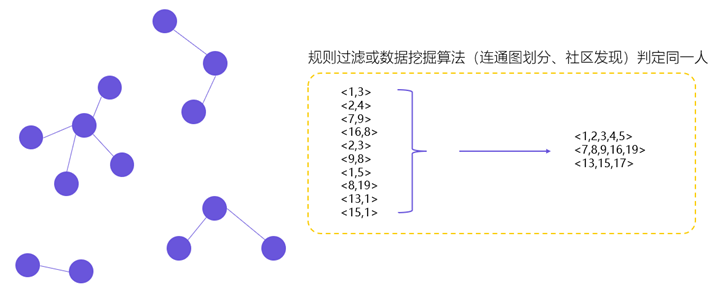# 选择的艺术：用数学获取最优选择

## 苏格拉底与麦穗问题• 第一个弟子没走多远，就看到一颗大麦穗，如获至宝地摘下。可是，越往前走，他越发现前面的麦穗远比手中的饱满。他懊恼而归。
• 第二个弟子吸取前者的教训，每看到一个大麦穗时，他总是收回了自己伸出去的手：更大的麦穗一定在前头。麦田快走完时，两手空空的弟子情知不妙，想采一颗，却又觉得最饱满的已经错过。他失望而归。
• 第三个弟子很聪明。他用前三分之一的路程去识别怎样的麦穗才是饱满的麦穗，第二个三分之一的路程去比较判断，在最后的三分之一的路程里他采摘了一颗最饱满的麦穗。他自然满意而归。

## 决策就是一次科学的赌博

1949 年，一位名为梅里尔·M. 弗勒德（Merrill M.Flood）的数学家首次提出了在学界赫赫有名的“未婚妻问题”。经过无数次的探讨、推导及演绎后，该问题广为流传的版本，便是著名的“波斯公主选驸马”难题。$$P(选中最优男)=P(当前男子为最优男) * P(被你选中｜当前男生为最优男)$$

• 最优男刚好位于样本空间s里，因为样本空间里的男生你已经全部无条件放弃了，所以这种情况你选中最优男的概率为0。
• 最优男刚好位于样本空间s的下一位，即位于s+1处。这时你100%能选中他，因为我们的策略就是只要遇见优于样本空间s里的人就做出选择。
• 最优男刚好位于样本空间s的下下一位，即位于s+2处。这时若要100%选中最优男，次优男必须位于样本空间s内（好于次优男的才是最优男）。而此时，次优男恰好位于样本空间是的概率为$\frac{s}{s+1}$（因为最优男在s+2处，所以次优男的位置只能是前面s+1的某处，所以位于样本空间s的概率就是$\frac{s}{s+1}$）。
• 最优男刚好位于样本空间s的下下下一位，即位于s+3处。这时若要100%选中最优男，次优男必须位于样本空间s内。而此时，次优男恰好位于样本空间是的概率为$\frac{s}{s+2}$（因为最优男在s+3处，所以次优男的位置只能是前面s+2的某处，所以位于样本空间s的概率就是$\frac{s}{s+2}$）。
• 最优男刚好位于样本空间s的下k位，即位于s+k处。这时若要100%选中最优男，次优男必须位于样本空间s内。而此时，次优男恰好位于样本空间是的概率为$\frac{s}{s+k-1}$
• 最优男处于最后一位，即末尾的n处。这时若要100%选中最优男，次优男必须位于样本空间s内。而此时，次优男恰好位于样本空间是的概率为$\frac{s}{n-1}$

$$P(被你选中｜当前男生为最优男)=1+ \frac{s}{s+1} + \frac{s}{s+2} + \frac{s}{s+3} +… + \frac{s}{s+k-1}+ … +\frac{s}{n-1}$$

$$P(选中最优男)=P(当前男生为最优男) * P(被你选中｜当前男生为最优男) \\ = \frac{1}{n} (1+ \frac{s}{s+1} + \frac{s}{s+2} + \frac{s}{s+3} +… + \frac{s}{s+k-1}+ … +\frac{s}{n-1}) \\ =\frac{s}{n}(1+ \frac{1}{s+1} + \frac{1}{s+2} + \frac{1}{s+3} +… + \frac{1}{s+k-1}+ … +\frac{s}{n-1}) \\= \frac{s}{n}\sum_{r=s}^{n-1}\frac{1}{r} \\= \frac{s}{n}\sum_{r=s}^{n-1}(\frac{n}{r} \cdot \frac{1}{n})$$

$$\frac{s}{n}\sum_{r=s}^{n-1}(\frac{n}{r} \cdot \frac{1}{n})\approx x\int_{x}^{1}\frac{1}{t} \mathrm{d}t=-x\ln x$$##### 网站与APP开发中的字体设置##### 用户体系搭建之ID-Mapping